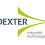## 05/10/2020

### Relation between Magnetic Flux density ( B ) and Magnetic Field Strength ( H )

Magnetic flux density ( B )

• It is defined as the magnetic flux per unit area of surface at right angle to the magnetic field. Its unit is weber per meter square

B = Φ / A

Where Φ = Flux in weber and

A = Surface area

Magnetic field intensity ( H )

• The magnetic field intensity is the force required to maintain magnetic flux and it will produces particular value of magnetic flux.
• The magnetic field strength is measure of magnetic field produced by electrical current flows through coil.
• The magnetic flux density is directly proportional to magnetic field intensity.

B α H

B = μH

Where μ = Constant

μ = μ0 μr

Where μ0 = Permeability of free space

= 4π × 10 – 7 weber / ampere - meter

μr  = Relative permeability of the medium

Relative Permeability of different Medium

 Medium Relative Permeability Air 1 Carbon steel 100 Electrical steel 4000 Super conductor 0

You may also like :

Constant Voltage Transformer

Constant Current Transformer

Earthing Transformer

1.2.3.